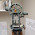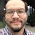## Wednesday, October 30, 2013

### Matrix Calculations: det(A - B * λ)=0

Let A and B be square matrices. B is a diagonal matrix. A diagonal matrix is a matrix with entries in it's diagonal positions, (1,1), (2,2), and so on, and 0 everywhere else. The identity matrix is a diagonal matrix. Another example is:

[ [2, 0, 0],[0, -2, 0],[0, 0, 5] ]

Let m, n, r, s, and t be numerical constants. Let a1, a2, and a3 be entries on the first row. Similar for b1, b2, b3, c1, c2, and c3.

det(A - B * ident(λ)) = 0

Comparison of 2 x 2 Matrices:
Note the constant term (λ^0), typed in green, the same.

B is a 2 x 2 identity matrix.

det( [ [a1, a2],[b1, b2] ] - λ * [ [1,0],[0,1] ] ) = 0
det( [ [a1, a2],[b1, b2] ] - [ [λ, 0],[0, λ] ]) = 0
λ^2 - (a1 + b2) * λ + a1*b2 - a2*b1 = 0

B is a diagonal matrix [ [m, 0],[0, n] ].

det( [ [a1, a2],[b1, b2] ] - λ * [ [m,0],[0,n] ] ) = 0
det( [ [a1, a2],[b1, b2] ] - [ [m*λ, 0],[0, n*λ] ]) = 0
m*n*λ^2 - (a1*n + b2*m)*λ + (a1*b2 - a2*b1) = 0

Comparison of 3 x 3 Matrices:
Note the constant term (λ^0), typed in green, the same.

B is a 3 x 3 identity matrix.

det( [ [a1,a2,a3],[b1,b2,b3],[c1,c2,c3] ] - λ * [ [1, 0, 0],[0, 1, 0],[0, 0, 1] ] ) = 0
det( [ [a1,a2,a3],[b1,b2,b3],[c1,c2,c3] ] - [ [λ, 0, 0],[0, λ, 0],[0, 0, λ] ] ) = 0

- λ^3
+ λ^2 * (a1 + b2 + c3)
+ λ * (-a1*b2 - a1*c3 - b2*c3 + a2*b1 + a3*c1 + b3*c2)
+ (a1*b2*c3 - a1*b3*c2 - a3*b2*c1 - a2*b1*c3 + a2*b3*c1 + a3*b1*c2) = 0

B is a general 3 x 3 diagonal matrix, [ [r, 0, 0],[0, s, 0],[0, 0, t] ].

det( [ [a1,a2,a3],[b1,b2,b3],[c1,c2,c3] ] - λ * [ [r, 0, 0],[0, s, 0],[0, 0, t] ] ) = 0
det( [ [a1,a2,a3],[b1,b2,b3],[c1,c2,c3] ] - [ [r*λ, 0, 0],[0, s*λ, 0],[0, 0, t*λ] ] ) = 0

- λ^3 * r*s*t
+ λ^2 * (s*t*a1 + r*t*b2 + r*s*c 3)
+ λ * (a1*b2*c3 - a1*b3*c2 - a3*b2*c1 - a2*b1*c3 + a2*b3*c1 + a3*b1*c2) = 0

Of course to get the eigenvalues (λ), solve the appropriate polynomial.

Hope this is helpful to those studying linear algebra or for those who are curious. This post was inspired from a program I was helping a fellow HP Prime programmer.

This post is dedicated to Michael de Estrada.

This blog is property of Edward Shore. 2013

1.1.